# Byte Battle

## Byte Battles

Byte Battles are a form of live coding, similar to Shader Showdowns, where two contestants compete in writing a visual effect in 25 minutes. The coding environment is the TIC-80 fantasy console. However, unlike Shader Showdowns, there is an additional limit: the final code should be 256 characters or less. This requires the contestants to use efficient code (e.g. single letter variables) and to minimize the code (e.g. remove the whitespace), all within the time limit. Unlike in normal TIC-80 sizecoding, there is no compression, so every character counts.

Symbol Meaning
`i` Pixel index
`s` Alias for math.sin
`x` Pixel x-coordinate
`y` Pixel y-coordinate

## Basic optimizations

• Functions, that are called three or more times should be aliased. For example, `e=elli` with `e()e()e()` is 3 characters shorter than `elli()elli()elli()`. Functions with 5-character-long names may already benefit from aliasing with two calls: `r=rectb` with `r()r()` is 1 character shorter than `rectb()rectb()`.
• `t=0` with `t=t+.01` is 1 character shorter than `t=time()*.6`.
• `for i=0,32639 do x=i%240y=i/240 end` is 2-3 characters shorter than `for y=0,135 do for x=0,239 do end end`.
• `(x*x+y*y)^.5` is 6 characters shorter than `math.sqrt(x*x+y*y)`.
• `s(w-11)` and `s(w+8)` both approximate `math.cos(w)`, so only `math.sin` needs to be aliased. `s(w-11)` is far more accurate, with the cost of one more character.

## One-lining

Most whitespace can be removed from LUA code. For example: `x=0y=0` is valid. All new lines can be removed or replaced with space, making the whole code a single line:

```function TIC()for i=0,32639 do poke4(i,i)end end
```

Warning: Letters `a-f` and `A-F` after a number cause problems. `a=0b=0` is not valid code. It is advisable to only used one letter variables in the ranges `g-z` and `G-Z` from the start; this will make eventual one-lining easier.

Function `load` takes a string of code and returns a function with no named arguments, with the code as its body. It's particularly useful for shortening the TIC function after one-lining:

```TIC=load'for i=0,32639 do poke4(i,i)end'
```

As a rule of thumb, one-lining and using the load trick can bring a ~ 275 character code down to 256.

Any arguments passes to a function defined with `load` can be fetched with the ellipsis (`...`). For example, `SCN=function(x)poke(16320,x)end` can be implemented as:

```SCN=load'poke(16320,...)'
```

This saves 6 characters. Multiple arguments can be fetched with `x,y=...`.

Warning: The backslash causes problems when using the load trick. In particular, if you have a string with escaped characters in the original code e.g. `print("foo\nbar")`, then this needs to be double-escaped: `load'print("foo\\nbar")'`

## Dithering

If you have a floating point color value, TIC-80 `pix` and `poke4` functions round it (toward zero). To add dithering, add a small value, between 0 and 1, to the color. The best technique depends whether you have `x` and `y` available or only `i` and how many bytes you can spare:

Expression Length Result Notes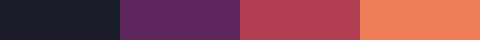No dithering
`i%.7` 4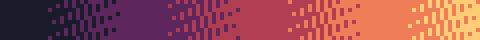`i^2.5%1` 7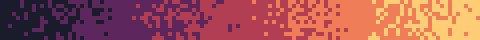"random" dithering
`s(i)*i%1` 8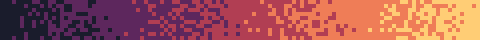also "random" dithering
`i*481/960%1` 11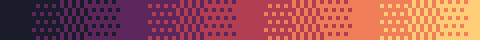`(x/2+y/4)%1` if you have x&y. `i*25/96%1` or `i*97/192` if desperate.
`(x*2-y%2)%4/4` 13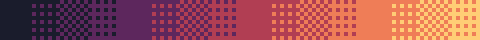2x2 block dithering
`(i*2-i//80%2)%4/4` 17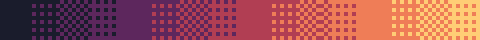2x2 block dithering (almost), from i only
`(x*2-y%2)%4/4+((x&2)-y//2%2)%4/16` 33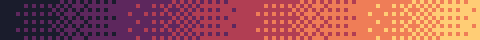4x4 with dithering matrix defined by an expression
`('0415627326158473'):sub(p,p)/8` 444x4 with data-defined dithering matrix, `p` defined as `p=y%4*4+x%4+1`

A quick example demonstrating the 2x2 block dithering:

```function TIC()
cls()
for i=0,2399 do
x=i%240
y=i//240
poke4(i,x/30+(x*2-y%2)%4/4)
end
end
```

## Palettes

The following palettes assume that `j` goes from 0 to 47. Usually there's no need to make a new loop for this: just reuse another loop with `j=i%48`.

Expression Length Result Notes
`poke(16320+j,j*5)` 17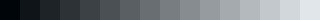`poke(16320+j,j%3*j*5)` 21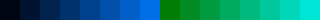Good for objects & background
`poke(16320+j,j%3*j/.4)` 22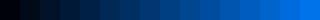Use `(j+1)%3`, `(j+2)%3` or `2*j%3` for different colors
`poke(16320+j,s(j)^2*255)` 24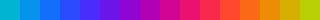Change the phase of the palette with s(j+p)
`poke(16320+j,s(j)^2*j*6)` 24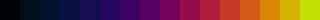Change the phase of the palette with s(j+p)
`poke(16320+j,s(j/15)*255)` 25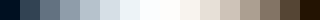`s(j/15)^2` is less bright
`poke(16320+j,s(j-j%-3)^2*255)` 29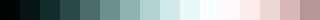`j%3*2` for a more blue/beige variant, `-j%3*4` for beige/blue variant
`poke(16320+j,255/(1+2^(4+j%3-j/5)))` 35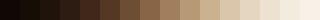`2*j%3` for a pink variant
`poke(16320+j,255/(1+2^(5-j%3-j/5)))` 35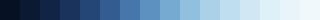`2*j%3` for a green variant
`poke(16320+j,s(j/15+s(j%3*3))^2*255)` 37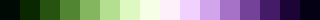Cyclic, based on 

The last one is an entire family of palettes. You can replace `s(j%3*3)` with any function that depends on `j%3`; this ensures the palette remains cyclic. Some ideas for tweaking the palettes:

• Invert the colors by adding a `-1-` in the expression
• Flip the blue/red channels & have the entire palette running backwards by using `poke(16367-j,...)`
• Abuse the default Sweetie 16 palette, by only setting some of the RGB channels, while keeping others as they are. For example, setting all the blue channels to zero: `poke(16322+j*3,0)`. Here `j` is between 0 and 15.

Code for testing palettes:

```function TIC()
cls()
for j=0,47 do poke(16320+j,s(j/15)*255)end
for c=0,15 do rect(c*5,0,5,5,c)end
end
s=math.sin
```

## Motion blur

In TIC-80 API, the `pix` and `poke4` functions round numbers towards zero. This can be abused for a motion blur: `poke4(i,peek4(i)-.9)` maps colors 1 to 15 into one lower value, but value 0 stays as it is. Like so:

```function TIC()
t=time()/9
circ(t%240,t%136,9,15)
for i=0,32639 do poke4(i,peek4(i)-.9)end
end
```

## Updating only some pixels

Pixel-based effects, especially raycasting and raymarching, can become excessively slow. A simple trick to update only ~ half of the pixels, giving a dithered/motion blur look and making the update smoother:

```function TIC()
t=time()/499
for i=t%2,32639,1.9 do poke4(i,i/4e3+t)end
end
```

## Examples of effects

The effects have not been crunched to keep them readable.

### Plasma

```function TIC()
t=time()/499
for i=0,32639 do
x=i%240
y=i/240
v=s(x/50+t)+s(y/22+t)+s(x/32)
poke4(i,v*2%8)
end
end
s=math.sin
```

### Rotozoomer

```function TIC()
t=time()/999
a=s(t-11)
b=s(t)
for i=0,32639 do
x=i%240-120
y=i/240-68
u=a*x-b*y
v=b*x+a*y
poke4(i,(u//1~v//1)//16)
end
end
s=math.sin
```

### Tunnel

```function TIC()
t=time()/199
for i=0,32639 do
x=i%240-s(t/7)*99-120
y=i/240-s(t/9)*49-68
u=math.atan2(y,x)*6/6.29
v=99/(x*x+y*y)^.5+t
poke4(i,u//1~v//1)
end
end
s=math.sin
```

### Raytracer

The raytraced geometry is a tunnel (cylinder). Note that the texture creates both positive and negative values, but applying the distance based fog to both values makes them darker, due to the way the palette is set.

```function TIC()
t=time()/199
for i=0,32639 do
-- set palette so scaling color based
-- on distance works
j=i%48
poke(16320+j,s(j/15)*255)
-- ray (u,v,w), not normalized!
u=i%240/120-1
v=i/32639-.5
w=1
-- rotate camera
a=s(t/23)*3
u,w=u*s(a+8)+w*s(a),w*s(a+8)-u*s(a)
-- find where ray hits tunnel wall
d=1/(u*u+v*v)^.5
z=d*w+t/9 -- move camera with time
q=math.atan2(u,v)
-- tunnel texture ("plasma")
c=s(q*5+s(z)*2)*s(z*2+s(q*3)*3)
poke4(i,c*.8^d*9) -- distance based fog
end
end
s=math.sin
```

### Raymarcher

The map is a bunch of repeated spheres here.

```function TIC()
for i=0,32639 do
-- ray (u,v,w), not normalized!
u=i%240/120-1
v=i/32639-.5
w=1
-- camera origo (x,y,z)
x=3
y=0
z=time()/999 -- camera moves with time
j=0
repeat
X=x%6-3 -- domain repetition
Y=y%6-3
Z=z%6-3
-- ray not normalized=>reduce scale
m=(X*X+Y*Y+Z*Z)^.5/2-1
x=x+m*u
y=y+m*v
z=z+m*w
j=j+1
until j>15 or m<.1
poke4(i,j)
end
end
```